Core Java - Interview Questions and Answers for 'Recursion' | Search Interview Question - javasearch.buggybread.com# Search Interview QuestionsMore than 3000 questions in repository.There are more than 900 unanswered questions.Have a video suggestion.
Click Correct / Improve and please let us know.
Label / Company      Label / Company / TextSubmit Question

Core Java - Interview Questions and Answers for 'Recursion' - 12 question(s) found - Order By Newest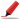Very frequently asked in HCL Tech ( Based of 4 inputs )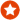Q1. Write a Program to reverse a string iteratively and recursively Core Java
Ans. Using String method -

new StringBuffer(str).reverse().toString();

Iterative -

Strategy - Loop through each character of a String from last to first and append the character to StringBuilder / StringBuffer

public static String getReverseString(String str){
StringBuffer strBuffer = new StringBuffer(str.length);
for(int counter=str.length -1 ; counter>=0;counter--){
strBuffer.append(str.charAt(counter));
}
return strBuffer;
}

Recursive -

Strategy - Call the method with substring starting from 2nd character recursively till we have just 1 character.

public static String getReverseString(String str){
if(str.length <= 1){
return str;
}
return (getReverseString(str.subString(1)) + str.charAt(0);
}Help us improve. Please let us know the company, where you were asked this question :LikeDiscussCorrect / Improvestring  StringBuffer  recursion  for loop  control statements  loop statement  stringbuffer.append  java.lang.String  java.lang.StringBuffer  String Manipulation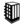Asked in 8 Companies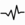frequentQ2. What will happen if we don't have termination statement in recursion ?
Ans. Function call allocates a stackframe in stack. Every stackframe will use some memory to store local variables, parameters and to remember return address. Without terminating condition stackframes will keep consuming memory from stack and eventually program will result in stackoverflow error.Help us improve. Please let us know the company, where you were asked this question :LikeDiscussCorrect / Improverecursion   break statement  break  stackoverflow exception  stackoverflow  memory management  memory  exceptionsQ3. Write a Program to print factorial of a number using recursion Core Java
public static void main(String args[]) {
System.out.println(factorial(5));
}

private static int factorial(int number){
if(number == 1){
return 1;
} else {
return number * factorial(number - 1);
}
}
}Help us improve. Please let us know the company, where you were asked this question :LikeDiscussCorrect / ImproverecursionAsked in 25 CompaniesQ4. Write a program to calculate factorial of a number using recursion Core Java
Ans.

public class Factorial {
public static void main(String[] args){
int x = 5;

System.out.println(calculateFactorial(x));

}

private static int calculateFactorial(int x){
if(x==1){
return 1;
}
return x * calculateFactorial(x-1);
}
}Help us improve. Please let us know the company, where you were asked this question :LikeDiscussCorrect / Improvefactorial  calculate factorial  code  coding  recursionAsked in 1 Companies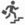basicQ5. Write an Algorithm for Graph Traversal ? The Graph has a loop. Algorithm
Ans. Please not that all such questions can be easily answered through recursion.

Simple recursive implementation could be

traverse(root);

void traverse(Element element){
if(element.hasNext()){
traverse(element.next());
} else {
System.out.println(element);
}
}

but this algo / code lead to endless loop if there is a loop in graph traversal.

So you can keep a collection to keep track of which elements have laready been traversed

static List<Elements> listOfAlreadyTraversedElements = new ArrayList<Elements>();

main(){
traverse(root);
}

void traverse(Element element){
if(element.hasNext()){
traverse(element.next());
} else {
System.out.println(element);
}
}Help us improve. Please let us know the company, where you were asked this question :LikeDiscussCorrect / Improvegraph traversal algorithm  graph traversal algorithm using recursionAsked in 1 CompaniesintermediateQ6. Write a program to print sum of numbers between the start and end number For example - Passing start number as 2 and end number as 10, it should print 2+3+4+5+6+7+8+9+10 = 54 Core Java
Ans.
public static void main(String args[]) {
System.out.println(sum(2,10));
}

private static int sum(int start,int end){
if(start > end){
return 0;
} else {
return start + sum(start+1,end);
}
}
}Help us improve. Please let us know the company, where you were asked this question :LikeDiscussCorrect / ImproverecursionQ7. Write a program using Recursion to print multiplication of numbers between the start and end number For example - Passing start number as 2 and end number as 10, it should print 2*3*4*5*6*7*8*9*10 = 3628800 Core Java
Ans.
public static void main(String args[]) {
System.out.println(multiply(2,5));
}

private static int multiply(int start,int end){
if(start > end){
return 1;
} else {
return start * multiply(start+1,end);
}
}
}Help us improve. Please let us know the company, where you were asked this question :LikeDiscussCorrect / ImproverecursionQ8. Write a Program to display numbers between 2 numbers using recursion Core Java
public static void main(String args[]) {
displayNumbersBetween(2,10);
}

private static void displayNumbersBetween(int start,int end){
if(start > end){
return ;
} else {
System.out.println(start);
displayNumbersBetween(start+1,end);
}
}
}Help us improve. Please let us know the company, where you were asked this question :LikeDiscussCorrect / Improverecursion  code  codingQ9. Write a Program to print asterix like following using Recursion ** *** **** ***** ****** ******* ******** ********* ********** Program should set the start and end index and then display this accordingly. For example the above pattern is for displayNumbersBetween(2,10) and following is for displayNumbersBetween(5,7) ***** ****** ******* Core Java
public static void main(String args[]) {
displayNumbersBetween(5,7);
}

private static void displayNumbersBetween(int start,int end){
if(start > end){
return ;
} else {
for(int x=1;x<=start;x++){
System.out.print("*");
}
System.out.println("");
displayNumbersBetween(start+1,end);
}
}
}Help us improve. Please let us know the company, where you were asked this question :LikeDiscussCorrect / Improverecursion  code  codingQ10. What will happen if we don't have termination statement in recursion ? Core Java
Ans. It would result in endless function calls and hence eventually would result in stackoverflow exception.Help us improve. Please let us know the company, where you were asked this question :LikeDiscussCorrect / Improverecursion  stackoverflowexceptionBasicfrequentQ11. Which of the two - iteration or recursion - is slower ? Core Java
Ans. Any function call is slower than the code in the same method as Java has to maintain stack of meta and function call information. So recursion is slower.Help us improve. Please let us know the company, where you were asked this question :LikeDiscussCorrect / Improveiteration  recursion  iteration vs recursionQ12. Write a Program to print fibonacci series using recursion. Core Java
Ans. public class FibonacciUsingRecursion {
public static void main(String[] args) throws IOException{
getNextFibanocci(1,1);
}

static int getNextFibanocci(int a,int b){
if(a+b >= 100){
return 0;
} else {
System.out.println(a+b);
getNextFibanocci(b,a+b);
}

return 0;
}
}Help us improve. Please let us know the company, where you were asked this question :LikeDiscussCorrect / Improvefibonacci  recursion

## Help us and Others Improve. Please let us know the questions asked in any of your previous interview.

Any input from you will be highly appreciated and It will unlock the application for 10 more requests.